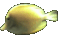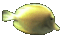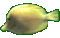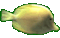Math Help - Vertex of Parabola Game - Use Maximum Point of Graph - Tips:

- A ball thrown upwards in still air follows an approximate parabolic arc.
- The curve h=-2(t-3)2+4 is a parabola that opens downwards and has a vertex at the point (3,4) .
- The curve h=3(t-4)2+20 is a parabola that opens upwards and has a vertex at the point (4,20) .

- The second degree (or quadratic) relation y=a(x-p)2+q represents a set of ordered pairs (x,y)
which form a parabolic shape having vertex (p,q) .

When 'a' is positive, the parabola opens upwards and the vertex is the lowest point.
When 'a' is negative, the parabola opens downwards and the vertex is the highest point.
Increasing the size of 'a', narrows the width of the mouth of the parabola.

- Your Game Score is reduced by the number of fish hits.

- To slow the game speed repeat tap/click on the word Slider.
- To increase the game speed repeat tap/click on the word Math.
- Speed can also be adjusted with a keyboard's - and + keys.

- Refresh/Reload the web page to restart the game.